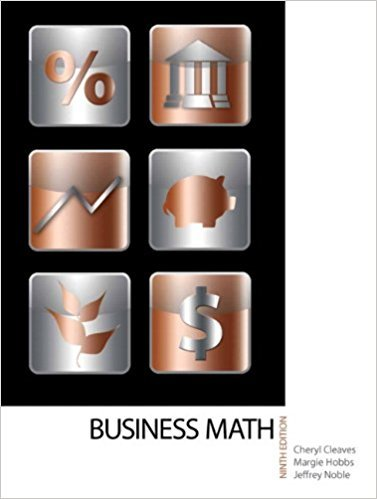×
×

# Solutions for Chapter 2-2: ADDING AND SUBTRACTING FRACTIONS## Full solutions for Business Math, | 9th Edition

ISBN: 9780135108178Solutions for Chapter 2-2: ADDING AND SUBTRACTING FRACTIONS

Solutions for Chapter 2-2
4 5 0 261 Reviews
21
1
##### ISBN: 9780135108178

Business Math, was written by and is associated to the ISBN: 9780135108178. Chapter 2-2: ADDING AND SUBTRACTING FRACTIONS includes 28 full step-by-step solutions. This textbook survival guide was created for the textbook: Business Math, , edition: 9. This expansive textbook survival guide covers the following chapters and their solutions. Since 28 problems in chapter 2-2: ADDING AND SUBTRACTING FRACTIONS have been answered, more than 19404 students have viewed full step-by-step solutions from this chapter.

Key Math Terms and definitions covered in this textbook
• Augmented matrix [A b].

Ax = b is solvable when b is in the column space of A; then [A b] has the same rank as A. Elimination on [A b] keeps equations correct.

• Basis for V.

Independent vectors VI, ... , v d whose linear combinations give each vector in V as v = CIVI + ... + CdVd. V has many bases, each basis gives unique c's. A vector space has many bases!

• Commuting matrices AB = BA.

If diagonalizable, they share n eigenvectors.

• Cyclic shift

S. Permutation with S21 = 1, S32 = 1, ... , finally SIn = 1. Its eigenvalues are the nth roots e2lrik/n of 1; eigenvectors are columns of the Fourier matrix F.

• Diagonalization

A = S-1 AS. A = eigenvalue matrix and S = eigenvector matrix of A. A must have n independent eigenvectors to make S invertible. All Ak = SA k S-I.

• Four Fundamental Subspaces C (A), N (A), C (AT), N (AT).

Use AT for complex A.

• Free columns of A.

Columns without pivots; these are combinations of earlier columns.

• Full column rank r = n.

Independent columns, N(A) = {O}, no free variables.

• Full row rank r = m.

Independent rows, at least one solution to Ax = b, column space is all of Rm. Full rank means full column rank or full row rank.

• Hessenberg matrix H.

Triangular matrix with one extra nonzero adjacent diagonal.

• Independent vectors VI, .. " vk.

No combination cl VI + ... + qVk = zero vector unless all ci = O. If the v's are the columns of A, the only solution to Ax = 0 is x = o.

• Multiplier eij.

The pivot row j is multiplied by eij and subtracted from row i to eliminate the i, j entry: eij = (entry to eliminate) / (jth pivot).

• Orthonormal vectors q 1 , ... , q n·

Dot products are q T q j = 0 if i =1= j and q T q i = 1. The matrix Q with these orthonormal columns has Q T Q = I. If m = n then Q T = Q -1 and q 1 ' ... , q n is an orthonormal basis for Rn : every v = L (v T q j )q j •

• Outer product uv T

= column times row = rank one matrix.

• Permutation matrix P.

There are n! orders of 1, ... , n. The n! P 's have the rows of I in those orders. P A puts the rows of A in the same order. P is even or odd (det P = 1 or -1) based on the number of row exchanges to reach I.

• Right inverse A+.

If A has full row rank m, then A+ = AT(AAT)-l has AA+ = 1m.

• Row space C (AT) = all combinations of rows of A.

Column vectors by convention.

• Singular matrix A.

A square matrix that has no inverse: det(A) = o.

• Volume of box.

The rows (or the columns) of A generate a box with volume I det(A) I.

• Wavelets Wjk(t).

Stretch and shift the time axis to create Wjk(t) = woo(2j t - k).

×